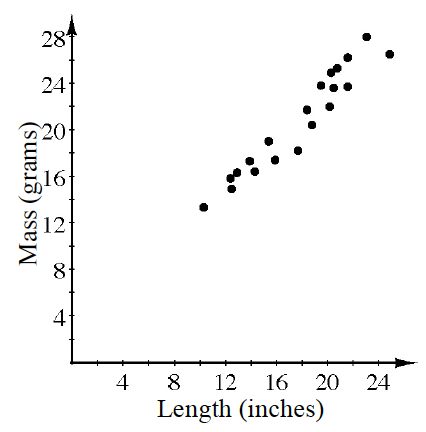### Home > GB8I > Chapter 3 Unit 4 > Lesson INT1: 3.3.2 > Problem3-128

3-128.

The graph at right shows a comparison of the length of several gold chain necklaces (including the clasp) to the total mass.

1. Write an equation for the line of best fit. What are the units for slope and y-intercept for your equation?

Eyeball a line of best fit. Then estimate the slope and $y$-intercept of your line.

$y = x + 2$

2. Based on your equation, what would you expect to be the mass of a $26$-inch chain?

• Substitute $26$ into your equation for $x$ and evaluate for $y$.